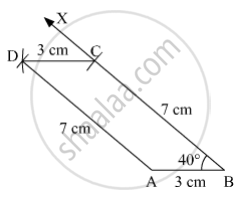SSC (English Medium) Class 8Maharashtra State Board
Share

# Construct a Parallelogram Abcd Such that L(Bc) = 7 Cm, M∠Abc = 40° , L(Ab) = 3 Cm. - SSC (English Medium) Class 8 - Mathematics

ConceptKinds of Quadrilaterals Concept of Parallelogram

#### Question

Construct a parallelogram ABCD such that l(BC) = 7 cm, m∠ABC = 40° , l(AB) = 3 cm.

#### Solution

Steps of Construction:

Step 1: Draw AB = 3 cm.

Step 2: Draw ∠ABX = 40° .

Step 3: With B as centre and radius 7 cm, draw an arc cutting the ray BX at C.

Step 4: With C as centre and radius 3 cm, draw an arc.

Step 5: With A as centre and radius 7 cm, draw an arc cutting the previous arc at D.

Step 6: Join AD and CD.Here, ABCD is the required parallelogram.

Is there an error in this question or solution?

#### APPEARS IN

Balbharati Solution for Balbharati Class 8 Mathematics (2019 to Current)
Chapter 8: Quadrilateral : Constructions and Types
Practice Set 8.3 | Q: 3 | Page no. 50
Solution Construct a Parallelogram Abcd Such that L(Bc) = 7 Cm, M∠Abc = 40° , L(Ab) = 3 Cm. Concept: Kinds of Quadrilaterals - Concept of Parallelogram.
S